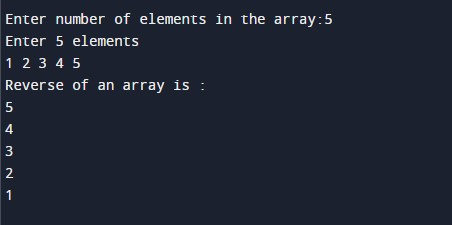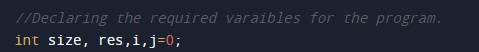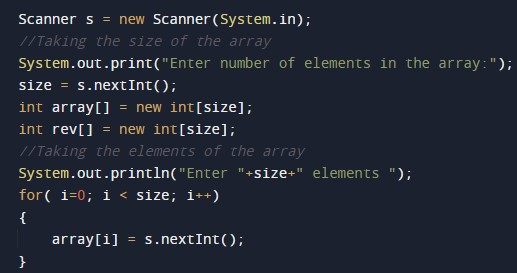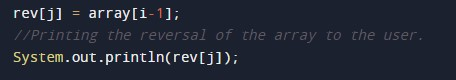# Java Program to print elements of an array in reverse order

In this tutorial you will learn about the Java Program to print elements of an array in reverse order and its application with practical example.

## Java Program to print elements of an array in reverse order

In this tutorial, we will learn to create a Java program that will print elements of an array in reverse order using Java programming.

## Prerequisites

Before starting with this tutorial, we assume that you are the best aware of the following Java programming topics:

• Operators in Java Programming.
• Basic Input and Output function in Java Programming.
• Basic Java programming.
• For loop in Java programming.
• Arithmetic operations in Java Programming.

## Reverse Order:-

In reverse order, we first take an array or declare an array, then reverse that array and print it.

Example:-

Array:- {1, 2, 3, 4, 5}

Reversed Array be:-{5, 4, 3, 2, 1}

## Program description to print elements of an array in reverse order.

In this program, First, we will first take the size of the array from the user. Then we will reverse the array using the for-loop and store it in a new variable. Then we will print the reverse of the original array to the user.

With the help of this program, we can print elements of an array in reverse order.

## Output:-In the above program, we have first initialized the required variable.• array[] = it will hold the integer value.
• i = it will hold the integer value.
• j = it will hold the integer value.
• size = it will hold the integer value.

Taking the size of the array and the elements.Program Code to Reverse an Array.Printing reversed array to the user.In this tutorial we have learn about the Java Program to print elements of an array in reverse order and its application with practical example. I hope you will like this tutorial.